### LARGE Function Examples in Excel, VBA, & Google Sheets

This tutorial demonstrates how to use the Excel LARGE Function in Excel to calculate the nth largest value.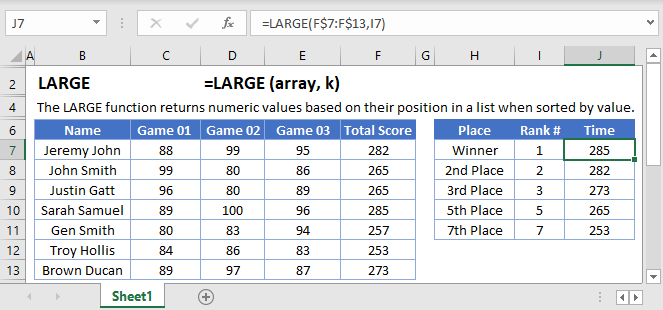## LARGE Function Overview

The LARGE Function Calculates the kth largest value.

To use the LARGE Excel Worksheet Function, select a cell and type: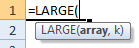(Notice how the formula inputs appear)

### LARGE function Syntax and inputs:

`=LARGE(array,k)`

array – An array of numbers.

k – The position of the value you want to return. Example: 6 would return the 6th largest value.

AutoMacro - VBA Code Generator

## How to use the LARGE Function

The LARGE Function returns the k-th largest number from a range of values. It is the opposite of the SMALL Function.

To use the LARGE function, use the following formula:

`=LARGE(B2:B9, 3)`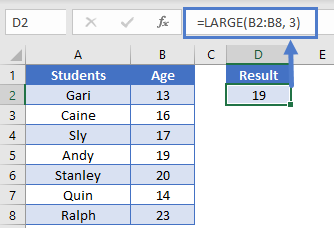Here we are looking for the 3rd largest number (3rd oldest student) in the range B2:B9.

### Out of Range Error

If you enter a number k, greater than the number of items in the range, the LARGE Function will return #NUM! error

`=LARGE(B2:B8, 8)`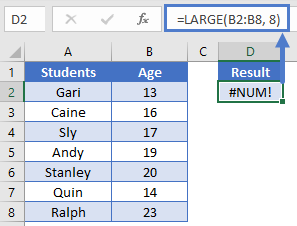Notice here we are looking for the 8th largest number in a range of only 7 numbers. So the LARGE Function returns #NUM!.

### Non-numeric Data

The LARGE Function will completely ignore non-numeric data as if that data is not part of the data range.

`=LARGE(B2:B8, 4)`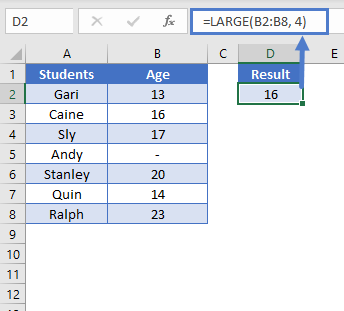## LARGE If

We can use the LARGE Function with criteria as well to perform a “large if”. For example, let’s say we want to find the 2nd oldest male student.

We will use this array formula:

`{=LARGE(IF(B2:B8 = "M", C2:C8), 2)}`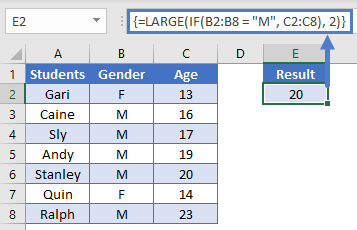Note: When building array functions, you must press CTRL + SHIFT + ENTER instead of just ENTER after creating your formula.

You’ll notice how the curly brackets appear. You can not just manually type in the curly brackets; you must use CTRL + SHIFT + ENTER.

To learn more about how the LARGE “IF” formula works, read our tutorial on LARGE If and LARGE IF.

## Sort with the LARGE Function

A quite useful use case for the LARGE Function is to sort data in descending (largest to smallest) order. To sort data, we combine the LARGE function with the ROW function.

`=LARGE(\$A\$2:\$A\$8, ROW()-1)`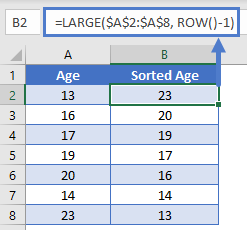This function works by using the ROW Function to define the k-value. Notice how row 2 has the largest number (k = 1), row 3 has the second largest (k = 2), so on & so forth. Thus, sorting the range from largest to smallest.

To learn more read our tutorial on Sorting with the SMALL and LARGE Functions.

Note: The above formula uses absolute references (the \$ signs) to lock cell references when copying formulas. If you aren’t familiar with this, please read our Excel References Guide (link to: https://www.automateexcel.com/excel/basics/cell-references?preview=true#Absolute_Frozen_and_Relative_References  )

## LARGE function in Google Sheets

The LARGE function works exactly the same in Google Sheets as in Excel.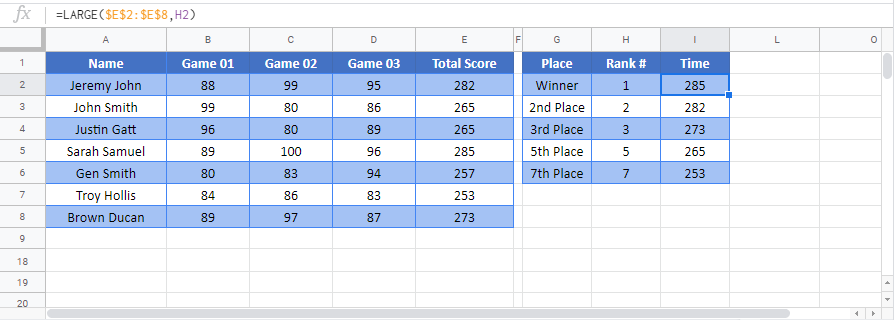## LARGE Examples in VBA

You can also use the LARGE function in VBA. Type:
`application.worksheetfunction.large(array,k)`
For the function arguments (array, etc.), you can either enter them directly into the function, or define variables to use instead.

Return to the List of all Functions in Excel

## Excel Practice Worksheet

Practice Excel functions and formulas with our 100% free practice worksheets!

• Automatically Graded Exercises
• Learn Excel, Inside Excel!